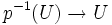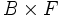# Bundle map

Jump to: navigation, search

This article defines a property of continuous maps between topological spaces

## Definition

A surjective continuous map$p:E \to B$ is termed a bundle map or fiber bundle with fiber$F$ (where$F$ is an abstract topological space) if the following is true:

• The fiber at any point is homeomorphic to$F$
• Every point in$B$ has an open neighbourhood$U$ such that the map$p^{-1}(U) \to U$ looks like the projection$U \times F \to U$ (this is called a local triviality condition)

If there is a homeomorphism from$E$ to$B \times F$ under which$p$ gets sent to the projection map, then we say that the bundle map is trivial.